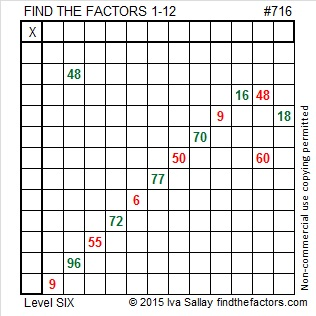# 716 and Level 6

• 716 is a composite number.
• Prime factorization: 716 = 2 x 2 x 179, which can be written 716 = (2^2) x 179
• The exponents in the prime factorization are 2 and 1. Adding one to each and multiplying we get (2 + 1)(1 + 1) = 3 x 2  = 6. Therefore 716 has exactly 6 factors.
• Factors of 716: 1, 2, 4, 179, 358, 716
• Factor pairs: 716 = 1 x 716, 2 x 358, or 4 x 179
• Taking the factor pair with the largest square number factor, we get √716 = (√4)(√179) = 2√179 ≈ 26.758176.You may find this Candy Cane puzzle more challenging than sweet:Print the puzzles or type the solution on this excel file: 12 Factors 2015-12-14

—————————————————————————————

Here are two more facts about the number 716:

86 + 87 + 88 + 89 + 90 + 91 + 92 + 93 = 716; that’s 8 consecutive numbers.

716 is palindrome 282 in BASE 17; note that 2(17²) + 8(17) + 2(1) = 716

—————————————————————————————This site uses Akismet to reduce spam. Learn how your comment data is processed.